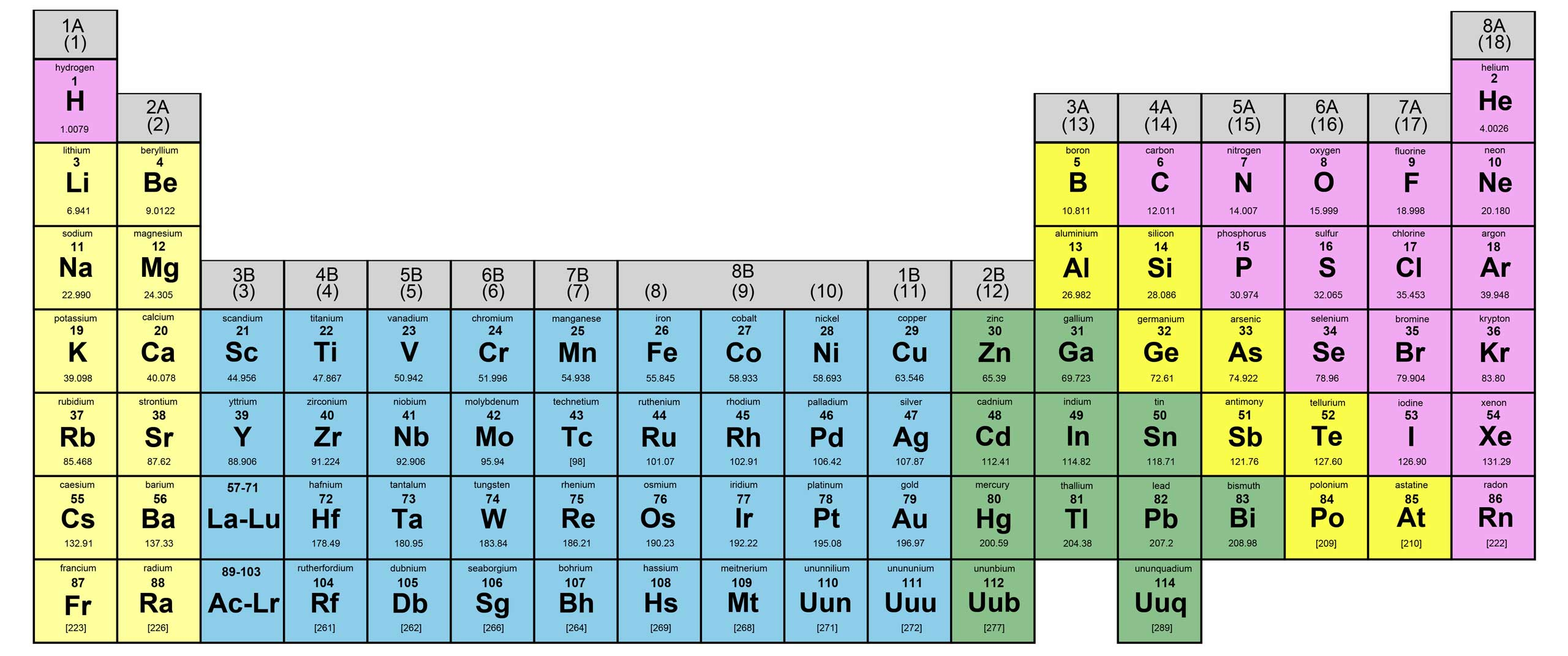Le Chatelier's PrincipleBackground  The chemical reaction will stay at chemical equilibrium until some other factor affect its condition. Regardless of how the system changes, there are only one of these two results: 1. The forward reaction speeds up to produce more product. 2. The reverse reaction speeds up to produce more reactant. After some time, the reaction will reach a new equilibrium point again. What is the Le Chatelier's principle? During the 1888, a French chemist Henri Louis Le Chatelier determined that the direction in which chemical equilibrium shifts can be figured out using a general principle.  Le Chatelier's principle - A change of conditions applied to a system in equilibrium will result a shift in the direction that best reduces the stress and a new equilibrium position will be reached. Let's look at some of the types of changes that affect equilibrium.1. Change in Concentration  If the concentration increases in the product or the reactant, the reaction will react faster for the side that got the increase. Example: If we add some N2 to the above reaction at equilibrium point, the forward reaction will increase in speed until a new equilibrium is reached. If the concentration decreases in the product or the reactant, the reaction will react faster on the side that did not get the decrease in the concentration. Example: If we remove some 2NH3 from the above reaction, the forward reaction will increase until a new equilibrium is reached. 2. Change in Volume If the volume increases, the reaction will shift in the direction that produces more moles of gas. Example: In this case, the reverse reaction will occur because the reactants have four total moles of gas vs. the two moles of gas in the product. If the volume decreases, the reaction will shift in the direction that produces fewer moles of gas. Example: In this case, the forward reaction will occur because the product has fewer moles of gas than the reactant. If the number of moles in the product and the reactant are the same, then nothing will happen. If there is no gas in the reaction, then nothing will happen. 3. Change in Temperature If the temperature increases: Example: We can look at the reactionthe same as. We can treat the Heat the same as another product, so if we increase the temperature it would be the same as increasing the concentration of the product. So the reaction will move in the reverse direction. A few other things will also effect the equilibrium point, such as the pressure change. However, it is very important to note that the addition of a catalyst will NOT change the equilibrium point.news | about us | contact us tutorials index | organic chemistry | practice tests | online quizzes | reference tools site copyright (c) 2002-2013 Learn Chem